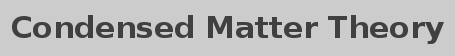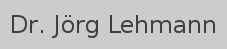Contact & Research | Publications | Talks

## Lecture (FS 2009): Random processes: Theory and applications from physics to finance

Random phenomena are of paramount importance in all areas of science. In the theory of random or stochastic processes, the random evolution of time-dependent quantities is considered. Prominent examples are the Brownian motion of a particle immersed in a liquid, the evolution of a chemical reaction, the motion of charge carriers in an electrical circuit, and the price fluctuations of a security on the stock market.

This lecture starts with a concise introduction to the basic concepts of probability theory and random processes. Markov processes as particularly important class of stochastic processes are discussed and their applications to various fields is presented.

### Topics

• Basics of probability theory
• Random processes: General concepts
• Markov processes:
• Master equation
• Fokker-Planck equation
• Stochastic differential equations
• Mathematical finance

### Time and place

Wednesday, 10:15-12:00h, Department of Physics, room 4.1

### Exercise classes

Exercise classes are held every Wednesday, 16:00-17:30h in room 4.1. The problem set which has been handed out the week before is discussed and solved together with the assistant Robert Andrzej Zak.

Per problem set, one problem has to be handed in and will be graded. Required for obtaining the credit points are 50% of the points of those problems and regular participation in the exercise class.

Uni Basel » Department of Physics » Condensed Matter Theory
last updated on 2008/03/19